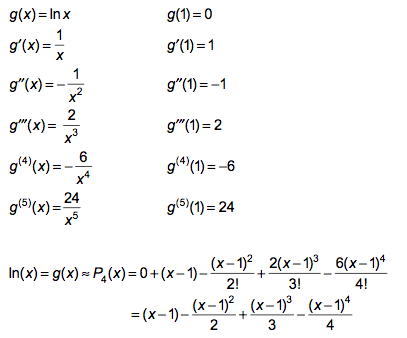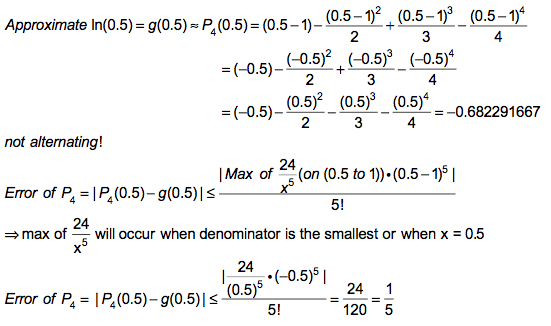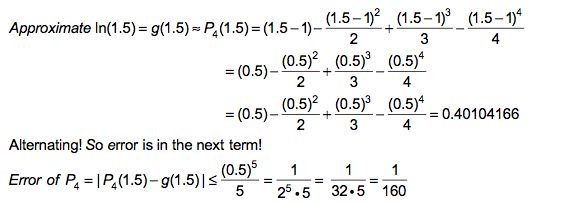# Taylor Series Polynomials

### 2. a) Find the P4 Taylor series for g(x) centered at x = 1 if  g(x) = ln(x)### b) Find approximation at x = ½ and compute the error.### c) Find approximation at x = 1.5 and compute the error.### c) Find approximation at x = 2 and compute the error.